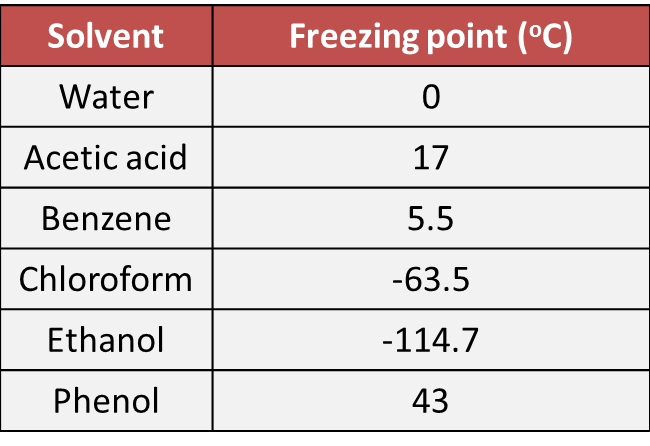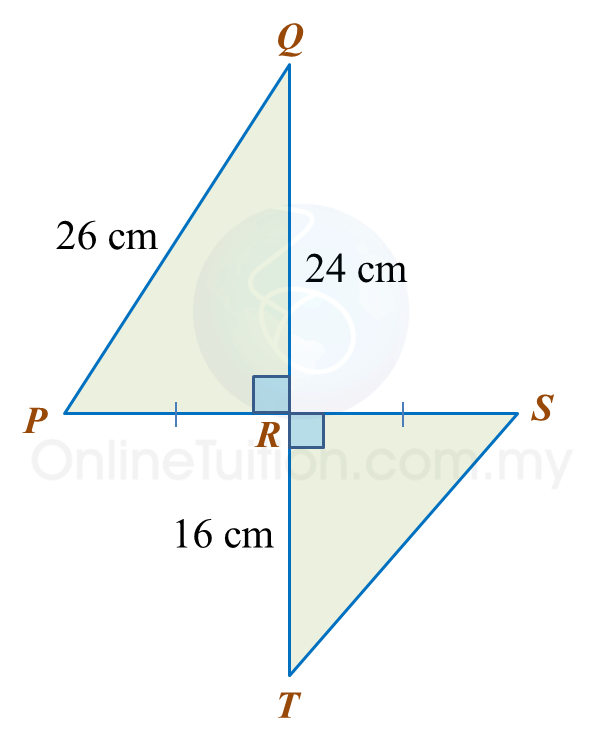# PT3 Mathematics 2017, Question 4

Question 4 (a):
Table 4 shows the freezing point of six solvents.(i) State the solvent which has the highest freezing point.
(ii) Find the difference in freezing points between benzene and chloroform.
(iii) State the number of solvents which freezing points are lower than acetic acid.

Solution:
(i)
Phenol

(ii)
Difference in freezing points between benzene and chloroform
= 5.5 – (–63.5)
= 69 oC

(iii)
Number of solvents which freezing points are lower than acetic acid = 4 (benzene, water, chloroform, ethanol).

Question 4 (b):
Diagram 4 shows two right angled triangles, PRQ and SRT. PRS is a straight line.Find the length, in cm of ST. Round off the answer to two decimal places.

Solution:

Question 4 (c):
It is given that  $\frac{2}{5}$ of Alex’s money is equal to  $\frac{1}{4}$ of Benny’s money.
If Benny has RM100, calculate the total amount of money for both of them.

Solution: